# RS Aggarwal Solutions for Class 7 Maths Exercise 6D Chapter 6 Algebraic Expressions

RS Aggarwal Solutions for Class 7 Maths Exercise 6D Chapter 6 Algebraic Expressions, Multiplication of two binomials is the topic covered in this exercise of RS Aggarwal Solutions for Class 7 Chapter 6. By using the distributive law of multiplication over addition twice, we may find their product. These RS Aggarwal Solutions for Class 7 Maths Chapter 6 Algebraic Expressions are extremely helpful for the students to clear all their doubts quickly.

## Download the PDF of RS Aggarwal Solutions For Class 7 Maths Chapter 6 Algebraic Expressions – Exercise 6D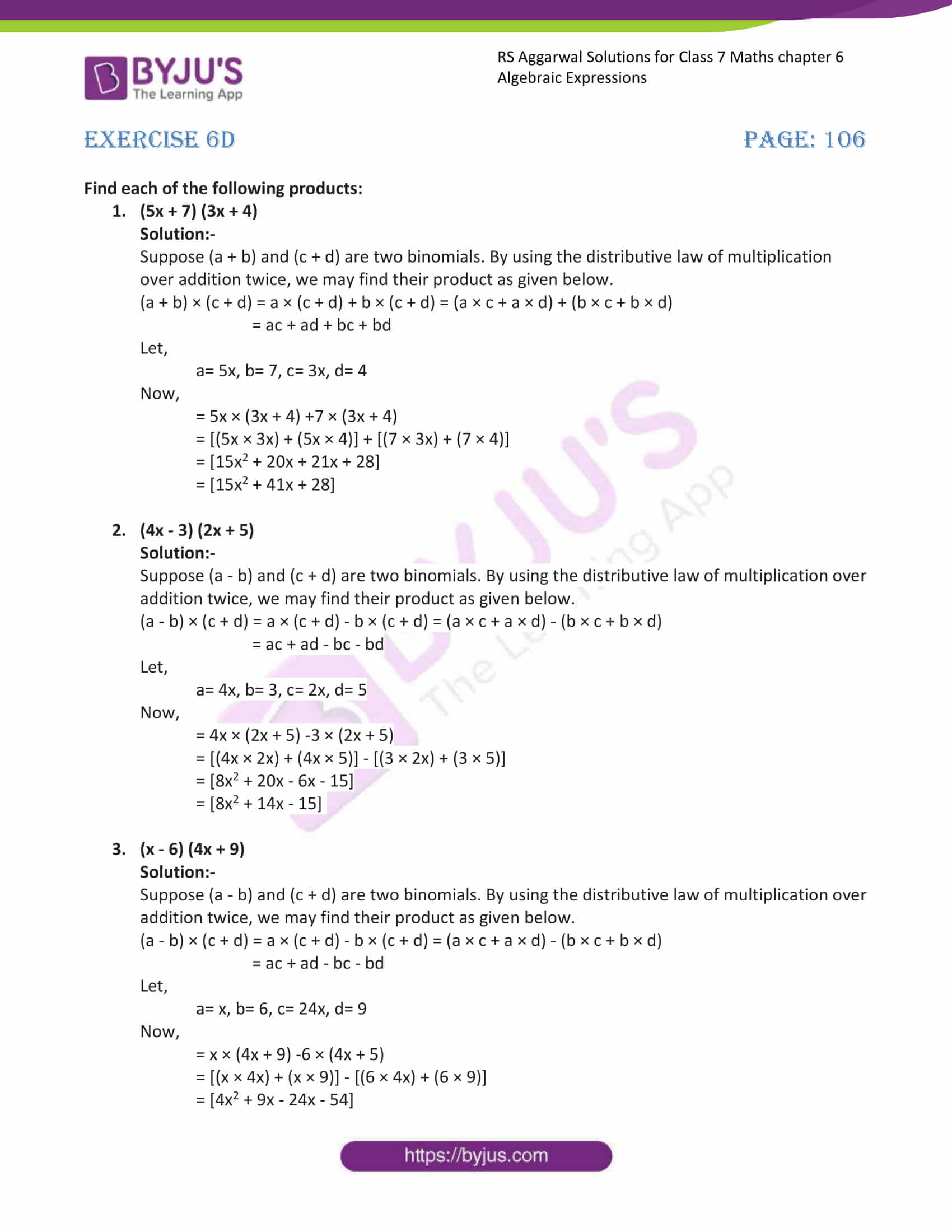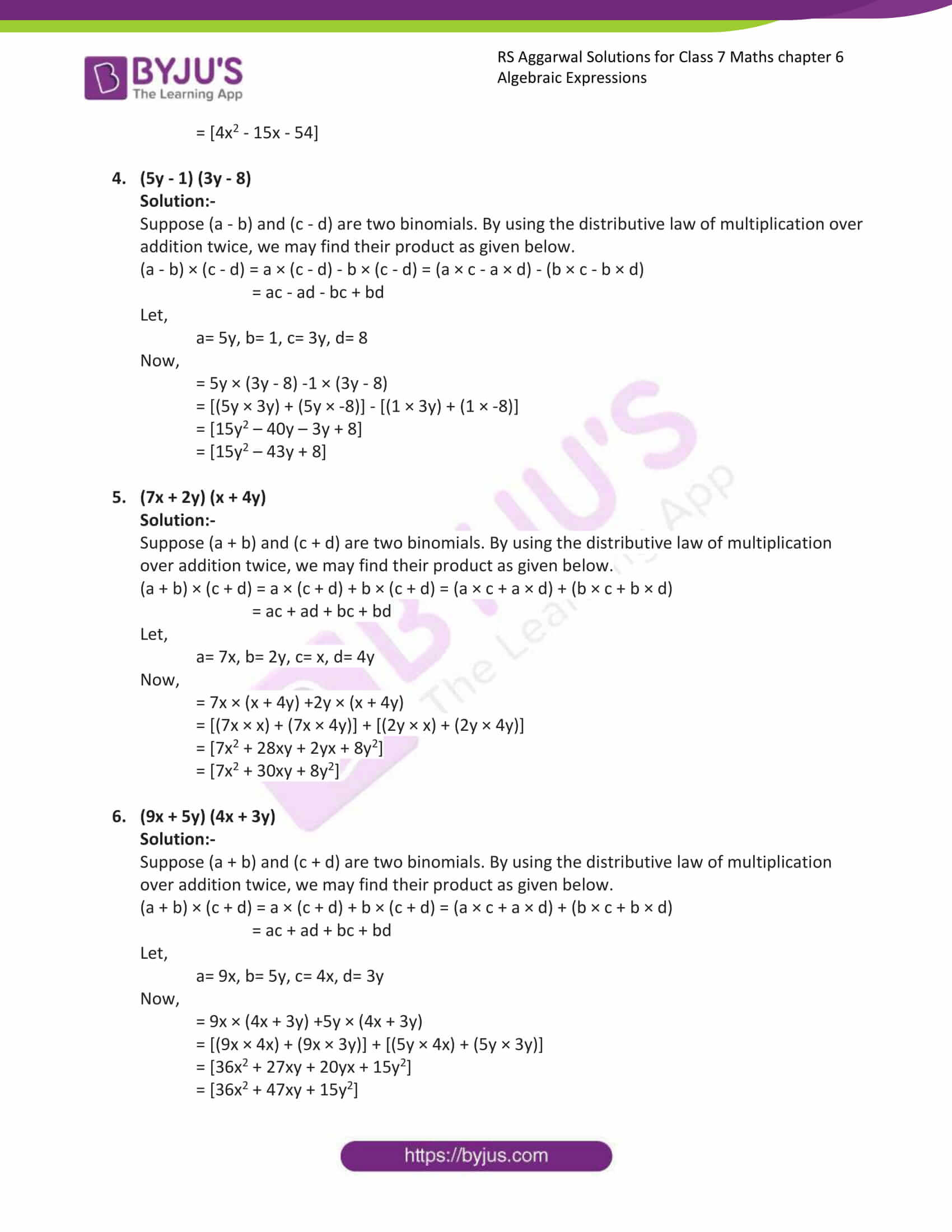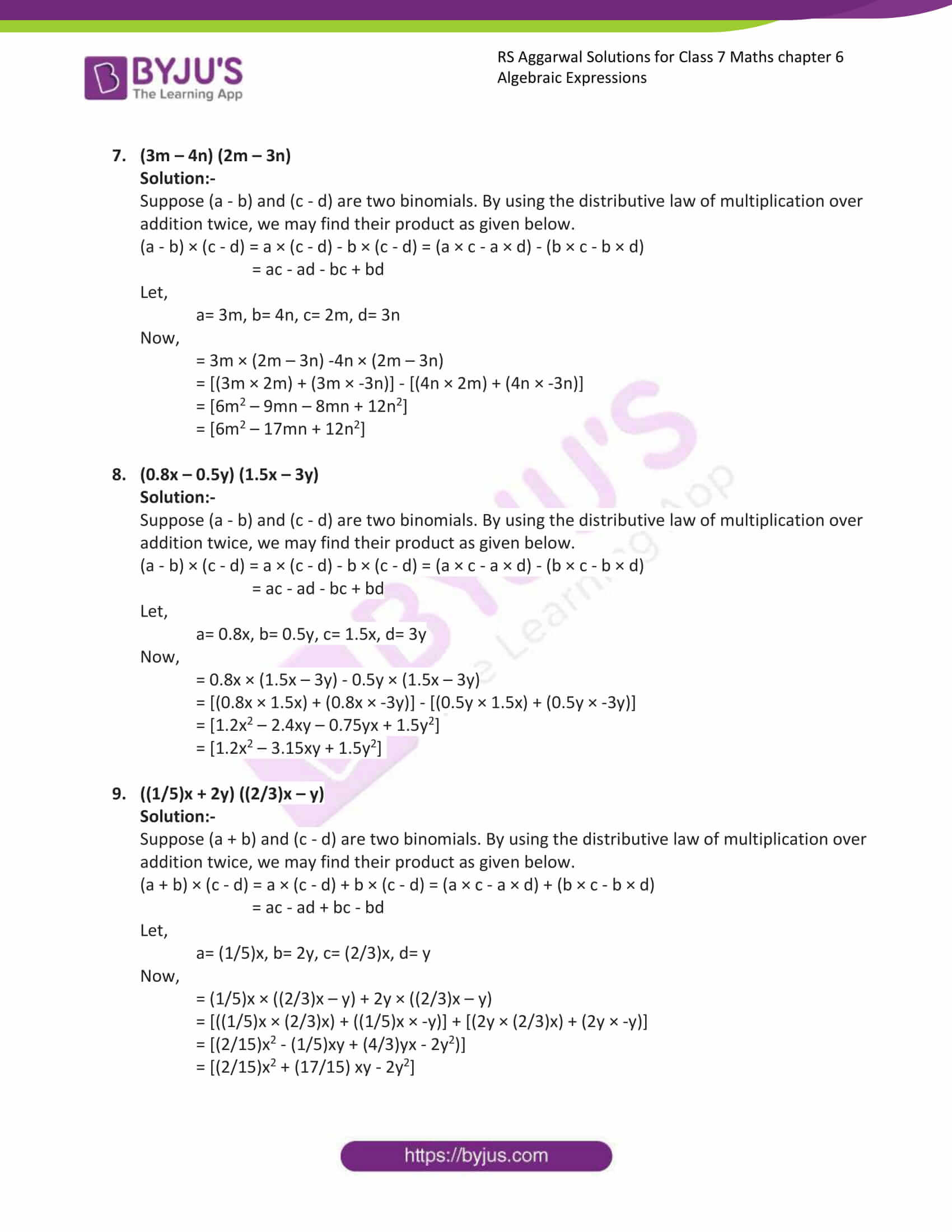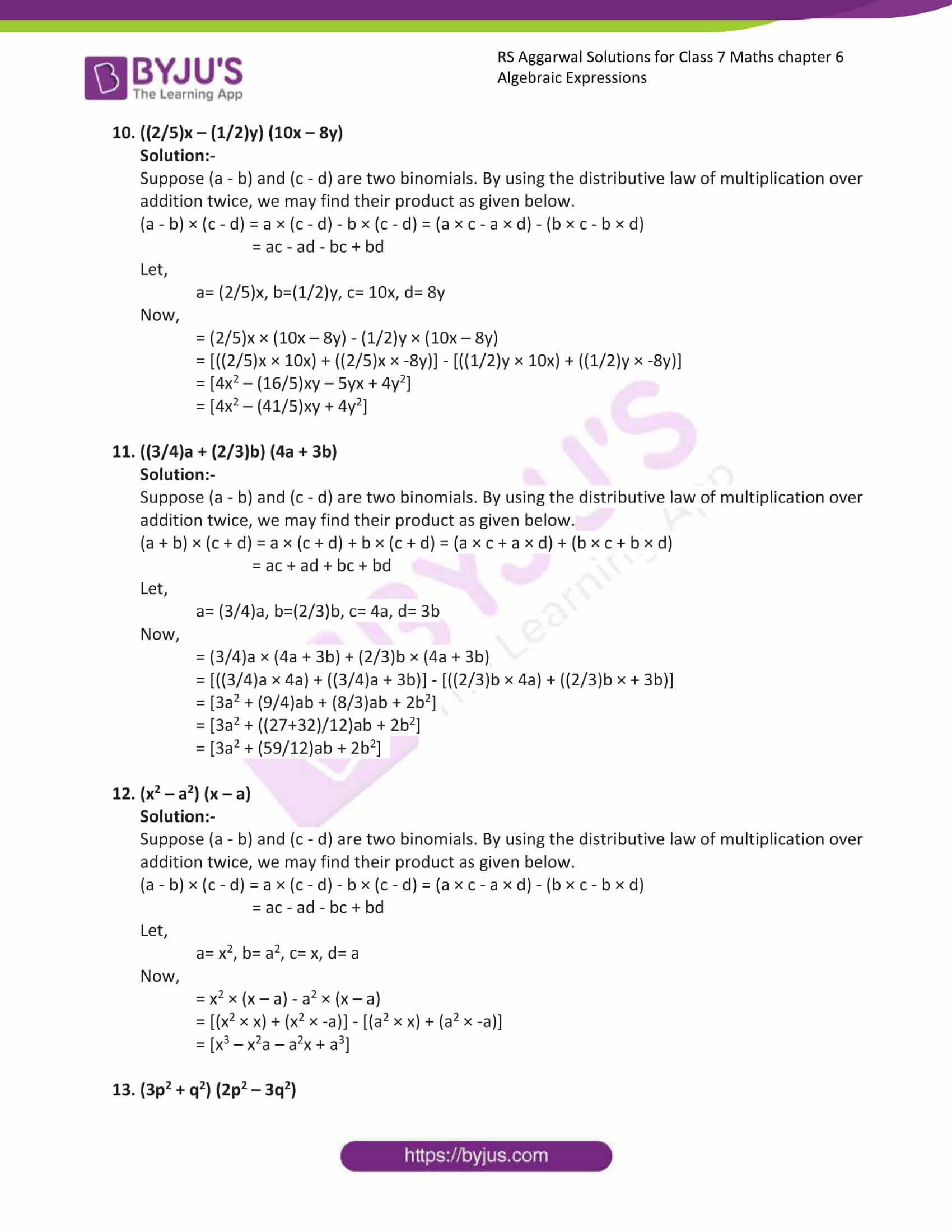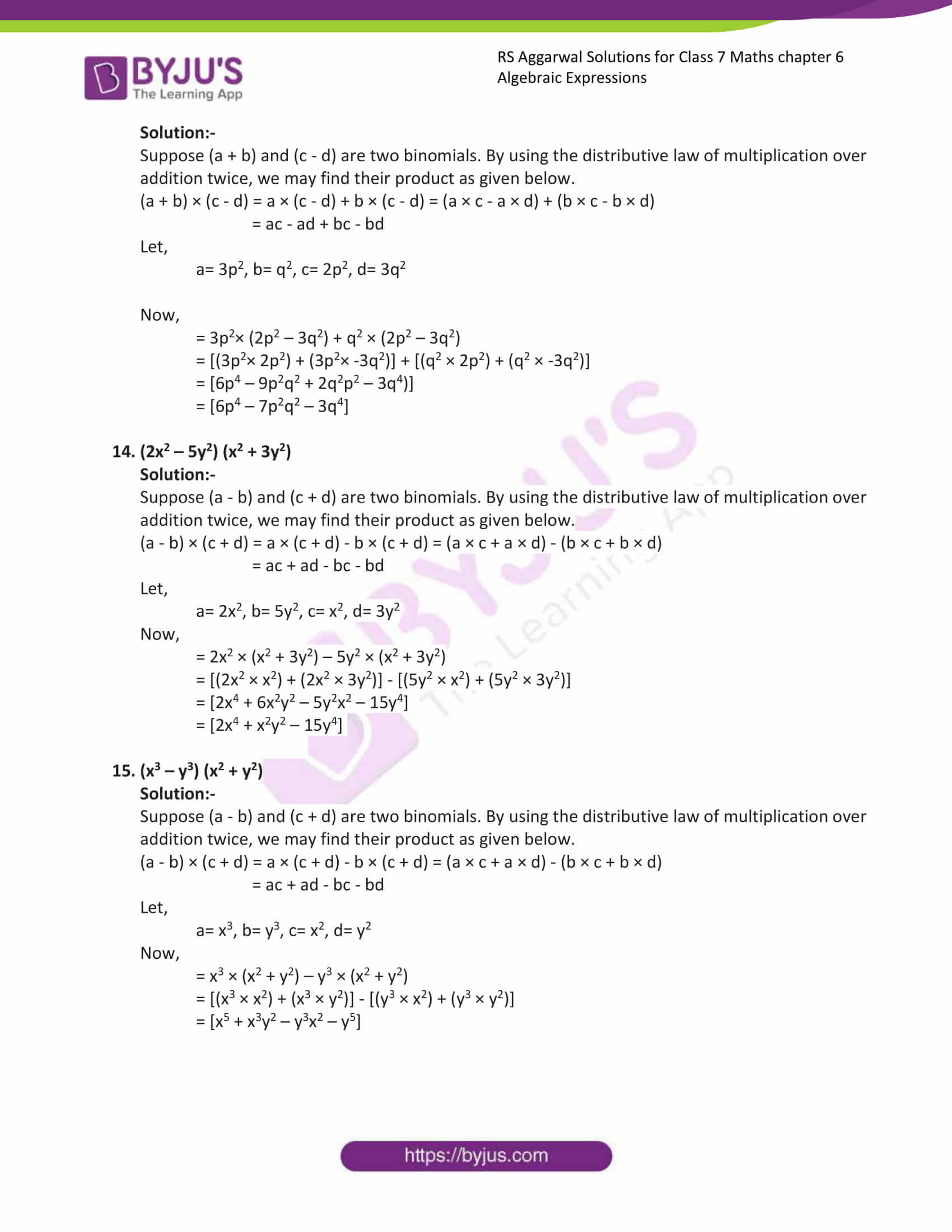### Access answers to Maths RS Aggarwal Solutions for Class 7 Chapter 6 – Algebraic Expressions Exercise 6D

Find each of the following products:

1. (5x + 7) (3x + 4)

Solution:-

Suppose (a + b) and (c + d) are two binomials. By using the distributive law of multiplication over addition twice, we may find their product as given below.

(a + b) × (c + d) = a × (c + d) + b × (c + d) = (a × c + a × d) + (b × c + b × d)

= ac + ad + bc + bd

Let,

a= 5x, b= 7, c= 3x, d= 4

Now,

= 5x × (3x + 4) +7 × (3x + 4)

= [(5x × 3x) + (5x × 4)] + [(7 × 3x) + (7 × 4)]

= [15x2 + 20x + 21x + 28]

= [15x2 + 41x + 28]

2. (4x – 3) (2x + 5)

Solution:-

Suppose (a – b) and (c + d) are two binomials. By using the distributive law of multiplication over addition twice, we may find their product as given below.

(a – b) × (c + d) = a × (c + d) – b × (c + d) = (a × c + a × d) – (b × c + b × d)

= ac + ad – bc – bd

Let,

a= 4x, b= 3, c= 2x, d= 5

Now,

= 4x × (2x + 5) -3 × (2x + 5)

= [(4x × 2x) + (4x × 5)] – [(3 × 2x) + (3 × 5)]

= [8x2 + 20x – 6x – 15]

= [8x2 + 14x – 15]

3. (x – 6) (4x + 9)

Solution:-

Suppose (a – b) and (c + d) are two binomials. By using the distributive law of multiplication over addition twice, we may find their product as given below.

(a – b) × (c + d) = a × (c + d) – b × (c + d) = (a × c + a × d) – (b × c + b × d)

= ac + ad – bc – bd

Let,

a= x, b= 6, c= 24x, d= 9

Now,

= x × (4x + 9) -6 × (4x + 5)

= [(x × 4x) + (x × 9)] – [(6 × 4x) + (6 × 9)]

= [4x2 + 9x – 24x – 54]

= [4x2 – 15x – 54]

4. (5y – 1) (3y – 8)

Solution:-

Suppose (a – b) and (c – d) are two binomials. By using the distributive law of multiplication over addition twice, we may find their product as given below.

(a – b) × (c – d) = a × (c – d) – b × (c – d) = (a × c – a × d) – (b × c – b × d)

= ac – ad – bc + bd

Let,

a= 5y, b= 1, c= 3y, d= 8

Now,

= 5y × (3y – 8) -1 × (3y – 8)

= [(5y × 3y) + (5y × -8)] – [(1 × 3y) + (1 × -8)]

= [15y2 – 40y – 3y + 8]

= [15y2 – 43y + 8]

5. (7x + 2y) (x + 4y)

Solution:-

Suppose (a + b) and (c + d) are two binomials. By using the distributive law of multiplication over addition twice, we may find their product as given below.

(a + b) × (c + d) = a × (c + d) + b × (c + d) = (a × c + a × d) + (b × c + b × d)

= ac + ad + bc + bd

Let,

a= 7x, b= 2y, c= x, d= 4y

Now,

= 7x × (x + 4y) +2y × (x + 4y)

= [(7x × x) + (7x × 4y)] + [(2y × x) + (2y × 4y)]

= [7x2 + 28xy + 2yx + 8y2]

= [7x2 + 30xy + 8y2]

6. (9x + 5y) (4x + 3y)

Solution:-

Suppose (a + b) and (c + d) are two binomials. By using the distributive law of multiplication over addition twice, we may find their product as given below.

(a + b) × (c + d) = a × (c + d) + b × (c + d) = (a × c + a × d) + (b × c + b × d)

= ac + ad + bc + bd

Let,

a= 9x, b= 5y, c= 4x, d= 3y

Now,

= 9x × (4x + 3y) +5y × (4x + 3y)

= [(9x × 4x) + (9x × 3y)] + [(5y × 4x) + (5y × 3y)]

= [36x2 + 27xy + 20yx + 15y2]

= [36x2 + 47xy + 15y2]

7. (3m – 4n) (2m – 3n)

Solution:-

Suppose (a – b) and (c – d) are two binomials. By using the distributive law of multiplication over addition twice, we may find their product as given below.

(a – b) × (c – d) = a × (c – d) – b × (c – d) = (a × c – a × d) – (b × c – b × d)

= ac – ad – bc + bd

Let,

a= 3m, b= 4n, c= 2m, d= 3n

Now,

= 3m × (2m – 3n) -4n × (2m – 3n)

= [(3m × 2m) + (3m × -3n)] – [(4n × 2m) + (4n × -3n)]

= [6m2 – 9mn – 8mn + 12n2]

= [6m2 – 17mn + 12n2]

8. (0.8x – 0.5y) (1.5x – 3y)

Solution:-

Suppose (a – b) and (c – d) are two binomials. By using the distributive law of multiplication over addition twice, we may find their product as given below.

(a – b) × (c – d) = a × (c – d) – b × (c – d) = (a × c – a × d) – (b × c – b × d)

= ac – ad – bc + bd

Let,

a= 0.8x, b= 0.5y, c= 1.5x, d= 3y

Now,

= 0.8x × (1.5x – 3y) – 0.5y × (1.5x – 3y)

= [(0.8x × 1.5x) + (0.8x × -3y)] – [(0.5y × 1.5x) + (0.5y × -3y)]

= [1.2x2 – 2.4xy – 0.75yx + 1.5y2]

= [1.2x2 – 3.15xy + 1.5y2]

9. ((1/5)x + 2y) ((2/3)x – y)

Solution:-

Suppose (a + b) and (c – d) are two binomials. By using the distributive law of multiplication over addition twice, we may find their product as given below.

(a + b) × (c – d) = a × (c – d) + b × (c – d) = (a × c – a × d) + (b × c – b × d)

= ac – ad + bc – bd

Let,

a= (1/5)x, b= 2y, c= (2/3)x, d= y

Now,

= (1/5)x × ((2/3)x – y) + 2y × ((2/3)x – y)

= [((1/5)x × (2/3)x) + ((1/5)x × -y)] + [(2y × (2/3)x) + (2y × -y)]

= [(2/15)x2 – (1/5)xy + (4/3)yx – 2y2)]

= [(2/15)x2 + (17/15) xy – 2y2]

10. ((2/5)x – (1/2)y) (10x – 8y)

Solution:-

Suppose (a – b) and (c – d) are two binomials. By using the distributive law of multiplication over addition twice, we may find their product as given below.

(a – b) × (c – d) = a × (c – d) – b × (c – d) = (a × c – a × d) – (b × c – b × d)

= ac – ad – bc + bd

Let,

a= (2/5)x, b=(1/2)y, c= 10x, d= 8y

Now,

= (2/5)x × (10x – 8y) – (1/2)y × (10x – 8y)

= [((2/5)x × 10x) + ((2/5)x × -8y)] – [((1/2)y × 10x) + ((1/2)y × -8y)]

= [4x2 – (16/5)xy – 5yx + 4y2]

= [4x2 – (41/5)xy + 4y2]

11. ((3/4)a + (2/3)b) (4a + 3b)

Solution:-

Suppose (a – b) and (c – d) are two binomials. By using the distributive law of multiplication over addition twice, we may find their product as given below.

(a + b) × (c + d) = a × (c + d) + b × (c + d) = (a × c + a × d) + (b × c + b × d)

= ac + ad + bc + bd

Let,

a= (3/4)a, b=(2/3)b, c= 4a, d= 3b

Now,

= (3/4)a × (4a + 3b) + (2/3)b × (4a + 3b)

= [((3/4)a × 4a) + ((3/4)a + 3b)] – [((2/3)b × 4a) + ((2/3)b × + 3b)]

= [3a2 + (9/4)ab + (8/3)ab + 2b2]

= [3a2 + ((27+32)/12)ab + 2b2]

= [3a2 + (59/12)ab + 2b2]

12. (x2 – a2) (x – a)

Solution:-

Suppose (a – b) and (c – d) are two binomials. By using the distributive law of multiplication over addition twice, we may find their product as given below.

(a – b) × (c – d) = a × (c – d) – b × (c – d) = (a × c – a × d) – (b × c – b × d)

= ac – ad – bc + bd

Let,

a= x2, b= a2, c= x, d= a

Now,

= x2 × (x – a) – a2 × (x – a)

= [(x2 × x) + (x2 × -a)] – [(a2 × x) + (a2 × -a)]

= [x3 – x2a – a2x + a3]

13. (3p2 + q2) (2p2 – 3q2)

Solution:-

Suppose (a + b) and (c – d) are two binomials. By using the distributive law of multiplication over addition twice, we may find their product as given below.

(a + b) × (c – d) = a × (c – d) + b × (c – d) = (a × c – a × d) + (b × c – b × d)

= ac – ad + bc – bd

Let,

a= 3p2, b= q2, c= 2p2, d= 3q2

Now,

= 3p2× (2p2 – 3q2) + q2 × (2p2 – 3q2)

= [(3p2× 2p2) + (3p2× -3q2)] + [(q2 × 2p2) + (q2 × -3q2)]

= [6p4 – 9p2q2 + 2q2p2 – 3q4)]

= [6p4 – 7p2q2 – 3q4]

14. (2x2 – 5y2) (x2 + 3y2)

Solution:-

Suppose (a – b) and (c + d) are two binomials. By using the distributive law of multiplication over addition twice, we may find their product as given below.

(a – b) × (c + d) = a × (c + d) – b × (c + d) = (a × c + a × d) – (b × c + b × d)

= ac + ad – bc – bd

Let,

a= 2x2, b= 5y2, c= x2, d= 3y2

Now,

= 2x2 × (x2 + 3y2) – 5y2 × (x2 + 3y2)

= [(2x2 × x2) + (2x2 × 3y2)] – [(5y2 × x2) + (5y2 × 3y2)]

= [2x4 + 6x2y2 – 5y2x2 – 15y4]

= [2x4 + x2y2 – 15y4]

15. (x3 – y3) (x2 + y2)

Solution:-

Suppose (a – b) and (c + d) are two binomials. By using the distributive law of multiplication over addition twice, we may find their product as given below.

(a – b) × (c + d) = a × (c + d) – b × (c + d) = (a × c + a × d) – (b × c + b × d)

= ac + ad – bc – bd

Let,

a= x3, b= y3, c= x2, d= y2

Now,

= x3 × (x2 + y2) – y3 × (x2 + y2)

= [(x3 × x2) + (x3 × y2)] – [(y3 × x2) + (y3 × y2)]

= [x5 + x3y2 – y3x2 – y5]

### Access other exercises of RS Aggarwal Solutions For Class 7 Chapter 6 – Algebraic Expressions

Exercise 6C Solutions